# Undersampling and Aliasing

August 8, 2019

As discussed in the previous lesson, sampling at less than the Nyquist rate is called undersampling. When multiple copies of the signal in the DTFT frequency domain overlap, it may cause what is known as aliasing. When aliasing occurs, a high-frequency component will take on the alias of a different low-frequency component.

### Example 7

#### Frequency Content of an Undersampled Waveform

Given the time waveform illustrated in Figure 2.1:

(1)The Fourier transform 𝖷̃(𝜔) is the triangle function illustrated in Figure 2.6, where the highest frequency component is 𝖿h = 1/2𝜋 Hz.

The waveform x(t) sampled at 𝖥s ≜ 1 × 𝖿h = 1/2𝜋 (giving a sample period of 𝜏 = 1/𝖥s = 2𝜋 ) is illustrated in Figure 2.9. This is an example of undersampling.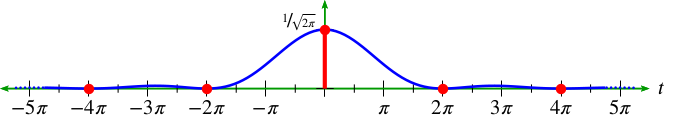Figure 2.9. An undersampled sequence.

All the samples are zero, except for the center sample at t=0. This will result in a DTFT this is constant across frequency 𝜔.

Using this sample rate and applying Theorem 1 will result in a DTFT 𝖸̃(𝜔) of y(n) that will overlap, or alias, in the DTFT’s frequency domain. It is expressed as:

(2)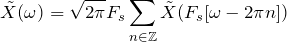(3)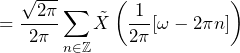### In conclusion:

• 𝖷̃(𝜔) repeats every 2𝜋 (this is true of all DTFTs)
• 𝖷̃(𝜔) reaches 0 at the place where (1/2𝜋) 𝜔 = 1…that is, at 𝜔 = 2𝜋
• The height of each triangle is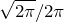or about 0.399
• The spectral content will simply be a constant, shown as the solid red line in Figure 2.10 below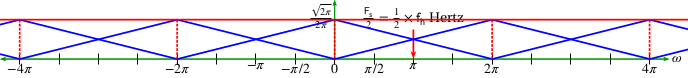Figure 2.10. Undersampled DTFT.